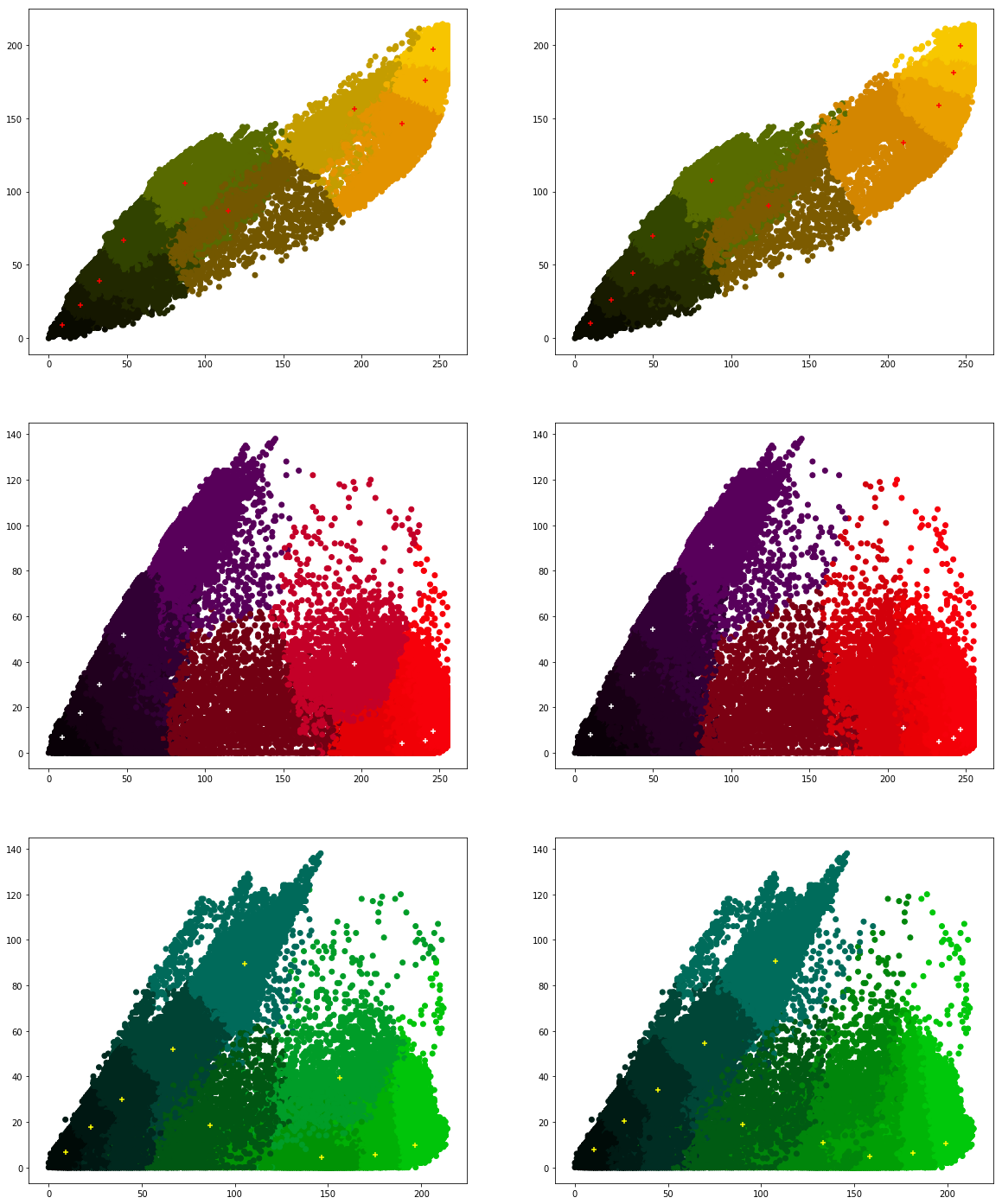### Goals

1. Plotting graphs using matplotlib
2. Reading and plotting image histograms.
3. Working with clustering and classification algorithms
4. Start working on recommender system project

#### Exercise 2.1 ★

matplotlib can be used to plot graphs. Given below is a very simple code with only x values. After importing the matplotlib library, we initialize x values and plot it.

```           ```
import matplotlib.pyplot as plot

x = [1, 2, 3, 4, 5, 6, 7, 8, 9, 10]
plot.plot(x)
plot.show()
```
```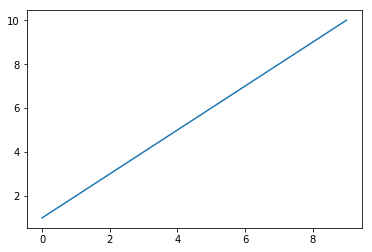Now let's change the color, style and width of the line.

```           ```
import matplotlib.pyplot as plot

x = [1, 2, 3, 4, 5, 6, 7, 8, 9, 10]
plot.plot(x, linewidth=3, drawstyle="steps", color="#00363a")
plot.show()
```
```

We will now initialize the y-values and plot the graph.

```           ```
import matplotlib.pyplot as plot

x = [1, 2, 3, 4, 5, 6, 7, 8, 9, 10]
y = [0, 1, 0, 0, 1, 0, 1, 1, 1, 0]
plot.plot(x, y, linewidth=3, drawstyle="steps", color="#00363a")
plot.show()
```
```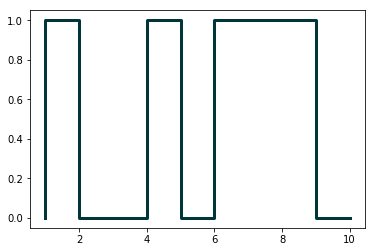In the first practical session, we saw how to parse JSON files. Continuing with the same JSON file, we will now plot the results of number of programming languages released per year. Verify the output.

```           ```
from pandas.io.json import json_normalize
import pandas as pd
import json
import matplotlib.pyplot as plot

dataframe = json_normalize(data)
grouped = dataframe.groupby('year').count()
plot.plot(grouped)
plot.show()
```
```

Following program will add title and labels to the x-axis and y-axis.

```           ```
from pandas.io.json import json_normalize
import pandas as pd
import json
import matplotlib.pyplot as plot

dataframe = json_normalize(data)
grouped = dataframe.groupby('year').count()
plot.plot(grouped)
plot.title("Programming languages per year")
plot.xlabel('year',  fontsize=16)
plot.ylabel('count',  fontsize=16)
plot.show()
```
```

There is yet another way to plot the dataframes, by using pandas.DataFrame.plot.

```           ```
from pandas.io.json import json_normalize
import pandas as pd
import json
import matplotlib.pyplot as plot

dataframe = json_normalize(data)

grouped = dataframe.groupby('year').count()
grouped = grouped.rename(
columns={'languageLabel':'count'}).reset_index()

grouped.plot(x=0, kind='bar', title="Programming languages per year")
```
```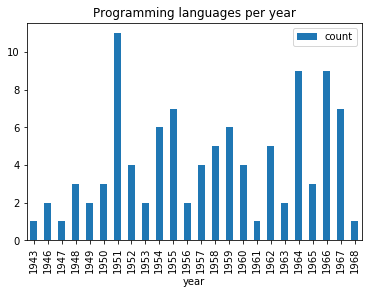Now, we want to create multiple subplots. A simple way is given below. Recall in first practical session, we did group by on multiple columns. Subplots can be used to visualize these data.

```           ```
from pandas.io.json import json_normalize
import pandas as pd
import json
import math
import matplotlib.pyplot as plot

array = []

for data in jsondata:
array.append([data['year'], data['languageLabel'],

dataframe = pd.DataFrame(array, columns=['year', 'languageLabel',
dataframe = dataframe.astype(dtype= {"year" : "int64",
"languageLabel" : "<U200",

grouped = grouped.rename(columns={'languageLabel':'count'})

#Initialization of subplots
nr = math.ceil(grouped.ngroups/2)
fig, axes = plot.subplots(nrows=nr, ncols=2, figsize=(20,25))

#Creation of subplots
for i, group in enumerate(grouped.groups.keys()):
g = grouped.get_group(group).reset_index()
g.plot(x='year', y='count', kind='bar',
title=group, ax=axes[math.floor(i/2),i%2])

plot.show()
```
```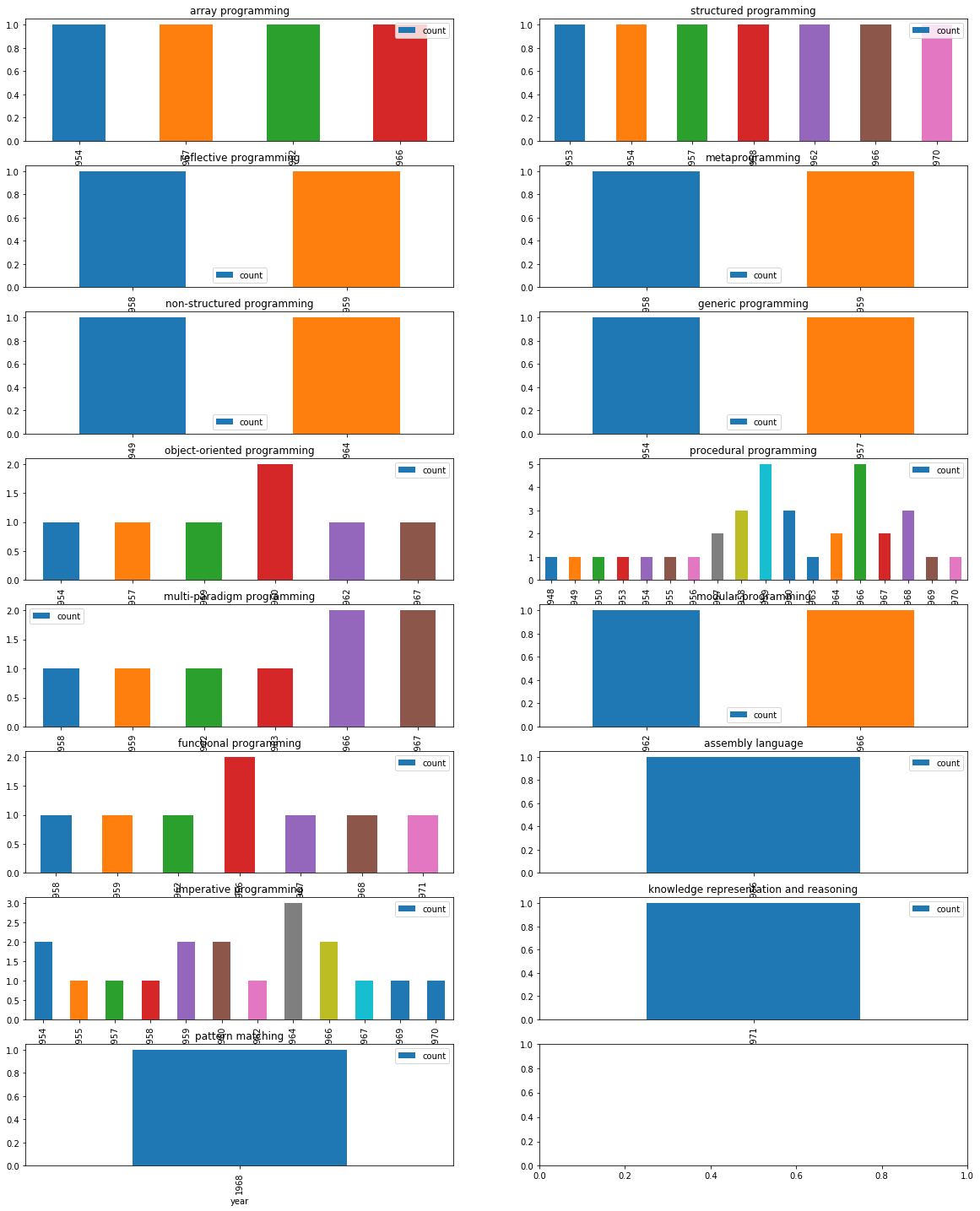Make changes to the above code, so that we can get visual information on count of languages of different programming paradigms released in every available year, i.e, for each year, we want to see the count of programming languages belonging to each programming language paradigm.

#### Exercise 2.2 ★

In this exercise, we will work on images. Download an image (e.g., picture.bmp and flower.jpg) in your current working folder and open it in the following manner. We will first try to get some metadata of the image.

```          ```
import os,sys
from PIL import Image
imgfile = Image.open("picture.bmp")
print(imgfile.size, imgfile.format)
```
```

We use Image module of Python PIL library (Documentation). We will now try to get data of 100 pixels from an image.

```          ```
import os,sys
from PIL import Image
imgfile = Image.open("flower.jpg")

data = imgfile.getdata()

for i in range(10):
for j in range(10):
print(i,j, data.getpixel((i,j)))
```
```

You may notice the pixel position and pixel values (a tuple of 3 values). Let's try to get additional metadata of the images, i.e., mode of image (e.g., RGB), number of bands, number of bits for each band, width and height of image (in pixels).

```          ```
import os,sys
from PIL import Image
imgfile = Image.open("flower.jpg")

print(imgfile.mode, imgfile.getbands(), imgfile.bits,
imgfile.width, imgfile.height)
```
```

Let's now get an histogram of colors. When you execute the following code, you will get a single array of values, frequency of each band (R, G, B etc.) concatenated together. In the following code, we will assume that we are working with an image of 3 bands (RGB mode) and each band is represented by 8 bits. We will plot the histogram of different colors.

```          ```
from PIL import Image
import matplotlib.pyplot as plot

imgfile = Image.open("flower.jpg")

histogram = imgfile.histogram()
# we have three bands (for this image)
red = histogram[0:255]
green = histogram[256:511]
blue = histogram[512:767]

fig, (axis1, axis2, axis3) = plot.subplots(nrows=3, ncols=1)
axis1.plot(red, color='red')
axis2.plot(green, color='green')
axis3.plot(blue, color='blue')
plot.show()
```
```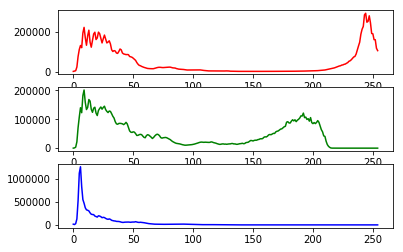But if wish to see all of them in one single plot.

```           ```
from PIL import Image
import matplotlib.pyplot as plot

imgfile = Image.open("flower.jpg")

histogram = imgfile.histogram()
red = histogram[0:255]
green = histogram[256:511]
blue = histogram[512:767]

x=range(255)

y = []
for i in x:
y.append((red[i],green[i],blue[i]))

plot.plot(x,y)
plot.show()
```
```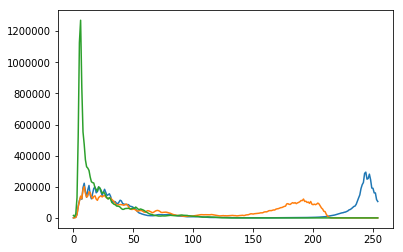But we do not wish to loose the band colors.

```           ```
from PIL import Image
import matplotlib.pyplot as plot

imgfile = Image.open("flower.jpg")

histogram = imgfile.histogram()
red = histogram[0:255]
green = histogram[256:511]
blue = histogram[512:767]

x=range(255)

y = []
for i in x:
y.append((red[i],green[i],blue[i]))

figure, axes = plot.subplots()
axes.set_prop_cycle('color', ['red', 'green', 'blue'])
plot.plot(x,y)
plot.show()
```
```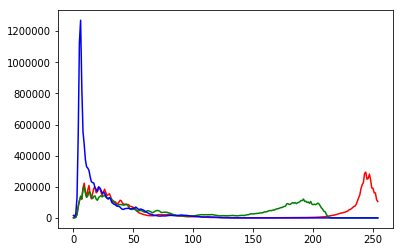Your next question is to get the top 20 intensities in each band and create a single plot of these top intensities. Write a python program that can achieve this.

#### Exercise 2.3 ★★

In this exercise, we will take a look at KMeans clustering algorithm. Continuing with images, we will now find 4 predominant colors in an image.

```           ```
from PIL import Image
import numpy
import math
import matplotlib.pyplot as plot
from sklearn.cluster import KMeans

imgfile = Image.open("flower.jpg")

numarray = numpy.array(imgfile.getdata(), numpy.uint8)

clusters = KMeans(n_clusters = 4)
clusters.fit(numarray)

npbins = numpy.arange(0, 5)
histogram = numpy.histogram(clusters.labels_, bins=npbins)
labels = numpy.unique(clusters.labels_)

barlist = plot.bar(labels, histogram)
for i in range(4):
barlist[i].set_color('#%02x%02x%02x' % (
math.ceil(clusters.cluster_centers_[i]),
math.ceil(clusters.cluster_centers_[i]),
math.ceil(clusters.cluster_centers_[i])))
plot.show()
```
```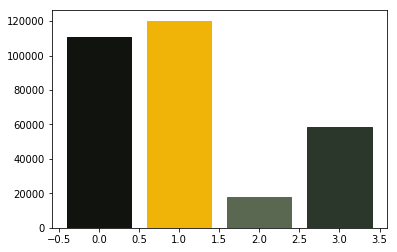For your next question, your goal is to understand the above code and achieve the following:

1. Assume that the number of clusters is given by the user, generalize the above code.
2. In case of bar chart, ensure that the bars are arranged in the descending order of the frequency of colors.
3. Also add support for pie chart in addition to the bar chart. Ensure that we use the image colors as the wedge colors. (e.g., given below)
4. Do you have any interesting observations?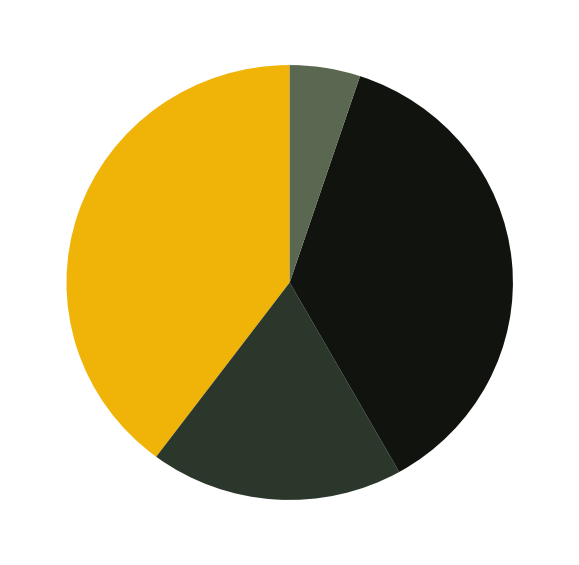#### Exercise 2.4 ★★

We will try to get more clusters and also check the time taken by each of these algorithms.

Let's start with some very simple exercises to experiment with the KMeans algorithm. Consider the following data and visualize it on a using a scatter plot.

```import numpy as np
import matplotlib.pyplot as plot

numarray = np.array([[1, 1], [1, 2], [1, 3], [1, 4], [1, 5],
[1, 6], [1, 7], [1, 8],[1, 9], [1, 10],
[10, 1], [10, 2], [10, 3], [10, 4], [10, 5],
[10, 6], [10, 7], [10, 8],[10, 9], [10, 10]])
plot.scatter(numarray[:, 0], numarray[:, 1])
plot.show()
```

Visually, it is quite evident that there are two clusters. But let's use KMeans algorithm to obtain the 2 clusters. We will first see the labels of our clustered data.

```import numpy as np
import matplotlib.pyplot as plot
from sklearn.cluster import KMeans

numarray = np.array([[1, 1], [1, 2], [1, 3], [1, 4], [1, 5],
[1, 6], [1, 7], [1, 8],[1, 9], [1, 10],
[10, 1], [10, 2], [10, 3], [10, 4], [10, 5],
[10, 6], [10, 7], [10, 8],[10, 9], [10, 10]])

clusters = KMeans(n_clusters = 2)
clusters.fit(numarray)
print(clusters.labels_)
```

Now, we will visualize the clusters using a scatter plot. We will use two colors for visually distinguishing them.

```import numpy as np
import matplotlib.pyplot as plot
from sklearn.cluster import KMeans

numarray = np.array([[1, 1], [1, 2], [1, 3], [1, 4], [1, 5],
[1, 6], [1, 7], [1, 8],[1, 9], [1, 10],
[10, 1], [10, 2], [10, 3], [10, 4], [10, 5],
[10, 6], [10, 7], [10, 8],[10, 9], [10, 10]])

clusters = KMeans(n_clusters = 2)
clusters.fit(numarray)
colors = np.array(["#ff0000", "#00ff00"])

plot.scatter(numarray[:, 0], numarray[:, 1], c=colors[clusters.labels_])
plot.show()
```

What if we tried to obtain 4 clusters? Try running the following code, multiple times. Any observation? Try changing the value of n_init with higher values.

```import numpy as np
import matplotlib.pyplot as plot
from sklearn.cluster import KMeans

numarray = np.array([[1, 1], [1, 2], [1, 3], [1, 4], [1, 5],
[1, 6], [1, 7], [1, 8],[1, 9], [1, 10],
[10, 1], [10, 2], [10, 3], [10, 4], [10, 5],
[10, 6], [10, 7], [10, 8],[10, 9], [10, 10]])

clusters = KMeans(n_clusters = 4, n_init=2)
clusters.fit(numarray)
colors = np.array(["#ff0000", "#00ff00", "#0000ff", "#ffff00"])

plot.scatter(numarray[:, 0], numarray[:, 1], c=colors[clusters.labels_])
plot.show()
```

Now we will try obtaining clusters with some real data (reference: citypopulation.json, Source: Wikidata). It contains information concerning different cities of the world: city name, year of its foundation and its population in the year 2010. In the following code, we want to cluster population data and to observe whether there is any correlation between age and recent population (2010) statistics. In the following code, there is a commented line. You can un-comment it to try with different population numbers. Any observation? Why did we use LabelEncoder? What is its purpose?

```from pandas.io.json import json_normalize
from sklearn.preprocessing import LabelEncoder
import pandas as pd
import json

dataframe = json_normalize(data)

le = LabelEncoder()
dataframe['cityLabel'] = le.fit_transform(dataframe['cityLabel'])
dataframe = dataframe.astype(dtype= {"year":"<i4",
"cityLabel":"<U200", "population":"i"})
dataframe = dataframe.loc[dataframe['year'] > 1500]
#dataframe = dataframe.loc[dataframe['population'] < 700000]
yearPopulation = dataframe[['year', 'population']]

clusters = KMeans(n_clusters = 2, n_init=1000)
clusters.fit(yearPopulation.values)
colors = np.array(["#ff0000", "#00ff00", "#0000ff", "#ffff00"])

plot.rcParams['figure.figsize'] = [10, 10]
plot.scatter(yearPopulation['year'], yearPopulation['population'],
c=colors[clusters.labels_])
plot.show()
```

Now let's continue working with flower.jpg. Let's start once again with KMeans and try to get clusters of size between 2 and 11.

```            ```
from PIL import Image
import numpy
import math
import matplotlib.pyplot as plot
from sklearn.cluster import KMeans

imgfile = Image.open("flower.jpg")
numarray = numpy.array(imgfile.getdata(), numpy.uint8)

X = []
Y = []

fig, axes = plot.subplots(nrows=5, ncols=2, figsize=(20,25))

xaxis = 0
yaxis = 0
for x in range(2, 12):
cluster_count = x

clusters = KMeans(n_clusters = cluster_count)
clusters.fit(numarray)

npbins = numpy.arange(0, cluster_count + 1)
histogram = numpy.histogram(clusters.labels_, bins=npbins)
labels = numpy.unique(clusters.labels_)

barlist = axes[xaxis, yaxis].bar(labels, histogram)
if(yaxis == 0):
yaxis = 1
else:
xaxis = xaxis + 1
yaxis = 0
for i in range(cluster_count):
barlist[i].set_color('#%02x%02x%02x' % (
math.ceil(clusters.cluster_centers_[i]),
math.ceil(clusters.cluster_centers_[i]),
math.ceil(clusters.cluster_centers_[i])))

plot.show()
```
```

Your next goal is to test the above code for cluster sizes between 2 and 21 which will give you the figure given below.

Note: The following image was generated after 6 minutes. Optionally, you can add print statements to test whether your code is working fine.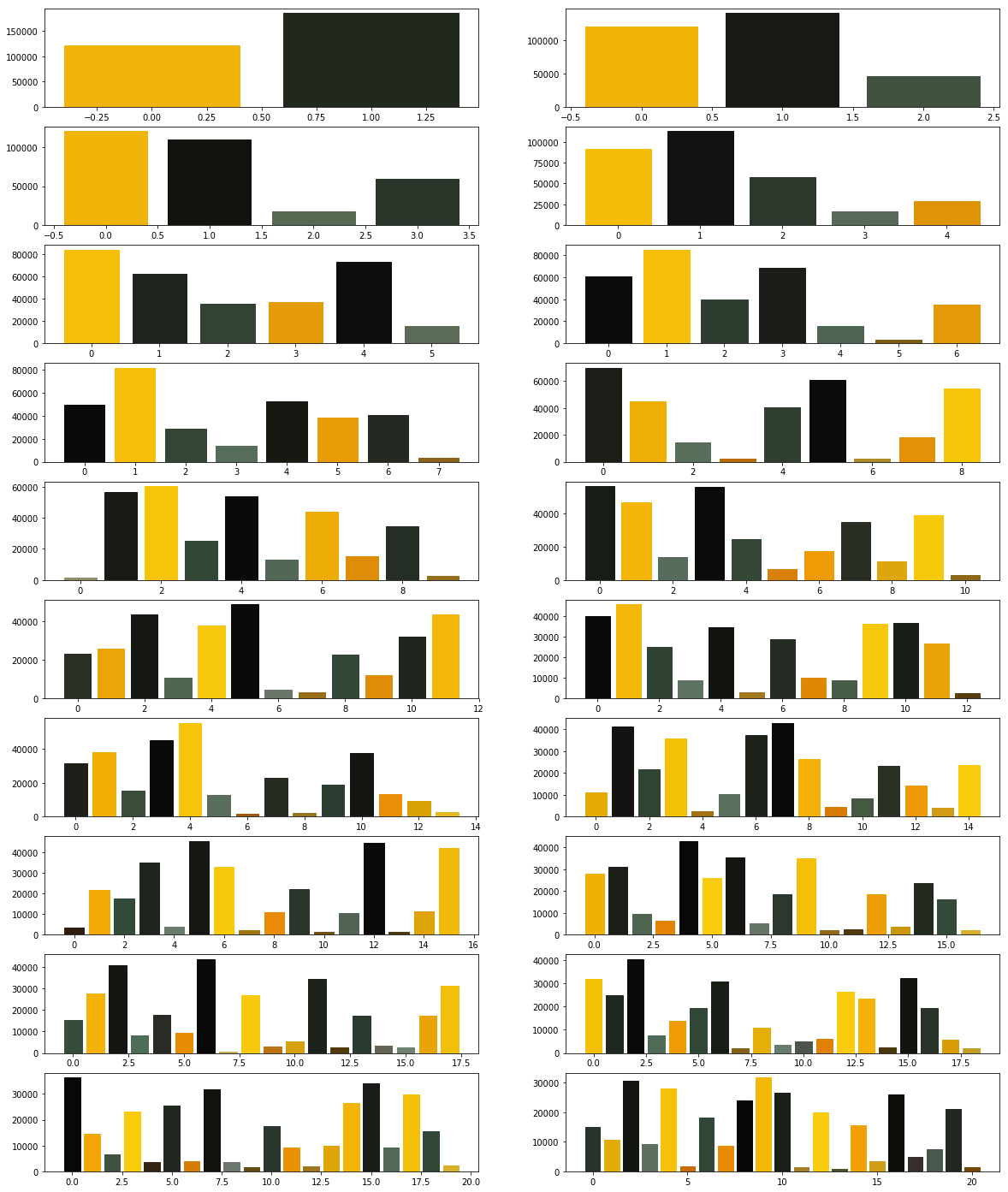Now we modify the above algorithm to use MiniBatchKMeans clustering algorithm (refer here). Observe the changes.

```            ```
from PIL import Image
import numpy
import math
import matplotlib.pyplot as plot
from sklearn.cluster import MiniBatchKMeans

imgfile = Image.open("flower.jpg")
numarray = numpy.array(imgfile.getdata(), numpy.uint8)

X = []
Y = []

fig, axes = plot.subplots(nrows=5, ncols=2, figsize=(20,25))

xaxis = 0
yaxis = 0
for x in range(2, 12):
cluster_count = x

clusters = MiniBatchKMeans(n_clusters = cluster_count)
clusters.fit(numarray)

npbins = numpy.arange(0, cluster_count + 1)
histogram = numpy.histogram(clusters.labels_, bins=npbins)
labels = numpy.unique(clusters.labels_)

barlist = axes[xaxis, yaxis].bar(labels, histogram)
if(yaxis == 0):
yaxis = 1
else:
xaxis = xaxis + 1
yaxis = 0
for i in range(cluster_count):
barlist[i].set_color('#%02x%02x%02x' % (
math.ceil(clusters.cluster_centers_[i]),
math.ceil(clusters.cluster_centers_[i]),
math.ceil(clusters.cluster_centers_[i])))

plot.show()
```
```

What did you observe? Your next goal is to test the above code for cluster sizes between 2 and 21 which will give you the figure given below.In order to compare the two algorithms, we consider the time taken by each of these algorithms. We will repeat the above experiment, but this time we will plot the time taken to obtain clusters of different sizes.

```            ```
from PIL import Image
import numpy
import math
import time
import matplotlib.pyplot as plot
from sklearn.cluster import KMeans

imgfile = Image.open("flower.jpg")
numarray = numpy.array(imgfile.getdata(), numpy.uint8)

X = []
Y = []

for x in range(1, 20):
cluster_count = x

start_time = time.time()
clusters = KMeans(n_clusters = cluster_count)
clusters.fit(numarray)
end_time = time.time()
total_time = end_time - start_time
print("Total time: ", x, ":", total_time)
X.append(x)
Y.append(total_time)

plot.bar(X, Y)
plot.show()
```
```

You may get a graph similar to the following.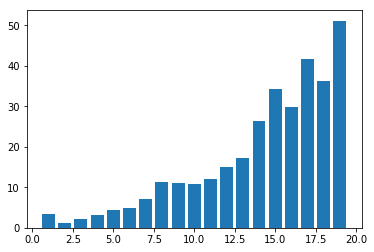We now use MiniBatchKMeans.

```            ```
from PIL import Image
import numpy
import math
import time
import matplotlib.pyplot as plot
from sklearn.cluster import MiniBatchKMeans

imgfile = Image.open("flower.jpg")
numarray = numpy.array(imgfile.getdata(), numpy.uint8)

X = []
Y = []

for x in range(1, 20):
cluster_count = x

start_time = time.time()
clusters = MiniBatchKMeans(n_clusters = cluster_count)
clusters.fit(numarray)
end_time = time.time()
total_time = end_time - start_time
print("Total time: ", x, ":", total_time)
X.append(x)
Y.append(total_time)

plot.bar(X, Y)
plot.show()
```
```

You may get a graph similar to the following.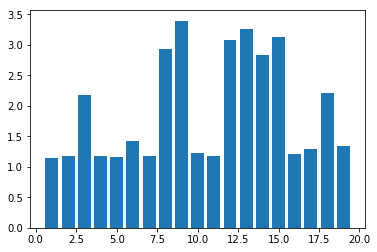Now test the above code using MiniBatchKMeans algorithm with cluster sizes between 2 and 50. What are your observations?

Finally we want to see whether we get the same cluster centers from both the algorithms. Run the following program to see the cluster centers produced by the two algorithms. We use two different colors (red and black) to distinguish the cluster centers from the two algorithms.

```            ```
from PIL import Image
import numpy
import math
import matplotlib.pyplot as plot
from sklearn.cluster import KMeans
from sklearn.cluster import MiniBatchKMeans

imgfile = Image.open("flower.jpg")
numarray = numpy.array(imgfile.getdata(), numpy.uint8)

cluster_count = 10

clusters = KMeans(n_clusters = cluster_count)
clusters.fit(numarray)

mclusters = MiniBatchKMeans(n_clusters = cluster_count)
mclusters.fit(numarray)

fig, axes = plot.subplots(nrows=3, ncols=1, figsize=(20,25))
#Scatter plot for RG (RGB)
axes.scatter(numarray[:,0],numarray[:,1])
axes.scatter(clusters.cluster_centers_[:,0],
clusters.cluster_centers_[:,1], c='red')
axes.scatter(mclusters.cluster_centers_[:,0],
mclusters.cluster_centers_[:,1], c='black')

#Scatter plot of RB (RGB)
axes.scatter(numarray[:,0],numarray[:,2])
axes.scatter(clusters.cluster_centers_[:,0],
clusters.cluster_centers_[:,2], c='red')
axes.scatter(mclusters.cluster_centers_[:,0],
mclusters.cluster_centers_[:,2], c='black')

#Scatter plot of GB (RGB)
axes.scatter(numarray[:,1],numarray[:,2])
axes.scatter(clusters.cluster_centers_[:,1],
clusters.cluster_centers_[:,2], c='red')
axes.scatter(mclusters.cluster_centers_[:,1],
mclusters.cluster_centers_[:,2], c='black')
```
```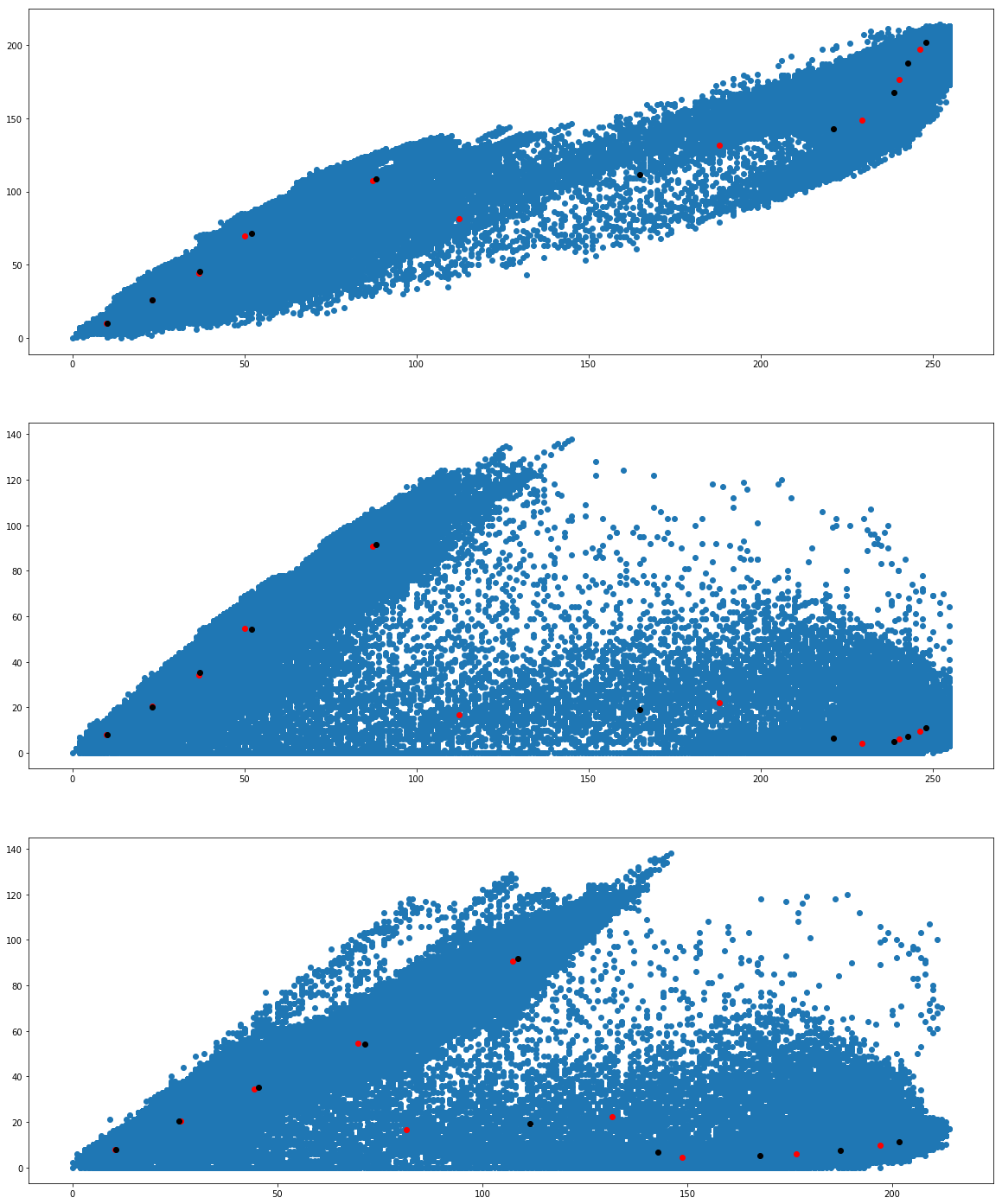We would like to see how the individual pixel values have been clustered. Run the following program a couple of times.

```            ```
from PIL import Image
import numpy
import math
import time
import matplotlib.pyplot as plot
from sklearn.cluster import KMeans
from sklearn.cluster import MiniBatchKMeans

imgfile = Image.open("flower.jpg")
numarray = numpy.array(imgfile.getdata(), numpy.uint8)

cluster_count = 10

mclusters = MiniBatchKMeans(n_clusters = cluster_count)
mclusters.fit(numarray)

npbins = numpy.arange(0, cluster_count + 1)
histogram = numpy.histogram(mclusters.labels_, bins=npbins)
labels = numpy.unique(mclusters.labels_)

fig, axes = plot.subplots(nrows=3, ncols=2, figsize=(20,25))

#Scatter plot for RG (RGB)
colors = []
for i in range(len(numarray)):
j = mclusters.labels_[i]
colors.append('#%02x%02x%02x' % (
math.ceil(mclusters.cluster_centers_[j]),
math.ceil(mclusters.cluster_centers_[j]), 0))

axes[0,0].scatter(numarray[:,0],numarray[:,1], c=colors)
axes[0,0].scatter(mclusters.cluster_centers_[:,0],
mclusters.cluster_centers_[:,1], marker="+", c='red')

#Scatter plot for RB (RGB)
colors = []
for i in range(len(numarray)):
j = mclusters.labels_[i]
colors.append('#%02x%02x%02x' % (
math.ceil(mclusters.cluster_centers_[j]),
0, math.ceil(mclusters.cluster_centers_[j])))

axes[1,0].scatter(numarray[:,0],numarray[:,2], c=colors)
axes[1,0].scatter(mclusters.cluster_centers_[:,0],
mclusters.cluster_centers_[:,2], marker="+", c='white')

#Scatter plot for GB (RGB)
colors = []
for i in range(len(numarray)):
j = mclusters.labels_[i]
colors.append('#%02x%02x%02x' % (0,
math.ceil(mclusters.cluster_centers_[j]),
math.ceil(mclusters.cluster_centers_[j])))

axes[2,0].scatter(numarray[:,1],numarray[:,2], c=colors)
axes[2,0].scatter(mclusters.cluster_centers_[:,1],
mclusters.cluster_centers_[:,2], marker="+", c='yellow')

clusters = KMeans(n_clusters = cluster_count)
clusters.fit(numarray)

npbins = numpy.arange(0, cluster_count + 1)
histogram = numpy.histogram(clusters.labels_, bins=npbins)
labels = numpy.unique(clusters.labels_)

#Scatter plot for RG (RGB)
colors = []
for i in range(len(numarray)):
j = clusters.labels_[i]
colors.append('#%02x%02x%02x' % (
math.ceil(clusters.cluster_centers_[j]),
math.ceil(clusters.cluster_centers_[j]), 0))

axes[0,1].scatter(numarray[:,0],numarray[:,1], c=colors)
axes[0,1].scatter(clusters.cluster_centers_[:,0],
clusters.cluster_centers_[:,1], marker="+", c='red')

#Scatter plot for RB (RGB)
colors = []
for i in range(len(numarray)):
j = clusters.labels_[i]
colors.append('#%02x%02x%02x' % (
math.ceil(clusters.cluster_centers_[j]),
0, math.ceil(clusters.cluster_centers_[j])))

axes[1,1].scatter(numarray[:,0],numarray[:,2], c=colors)
axes[1,1].scatter(clusters.cluster_centers_[:,0],
clusters.cluster_centers_[:,2], marker="+", c='white')

#Scatter plot for GB (RGB)
colors = []
for i in range(len(numarray)):
j = clusters.labels_[i]
colors.append('#%02x%02x%02x' % (0,
math.ceil(clusters.cluster_centers_[j]),
math.ceil(clusters.cluster_centers_[j])))

axes[2,1].scatter(numarray[:,1],numarray[:,2], c=colors)
axes[2,1].scatter(clusters.cluster_centers_[:,1],
clusters.cluster_centers_[:,2], marker="+", c='yellow')
plot.show()
```
```